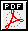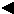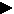About  PDFFull Text(15 K)
 Weeds and Herbicides in Arizona: Surveys of Plant Populations and Grower Practices William B. McCloskey, Paul B. Baker and Will Sherman ABSTRACT Cotton fields were surveyed for weeds in 1995 late in the season, either immediately before harvest or after harvest using the quantitative survey method of Thomas (1985, Weed Sci. vol. 33:34-43). The 82 fields surveyed across the state were randomly selected and were a subset of the fields used by the USDA-Arizona Agricultural Statistics Service to determine objective cotton yield estimates during the season. An inverted "W" pattern was used to systematically walk each sample field. Each leg or transect on the inverted "W" contained 5 equally spaced quadrats for a total of 20 quadrats per field. Each quadrat consisted of 2 adjacent cotton rows by 10 feet of row. With a statewide average row spacing of 37.47 inches, an average of 116 m2 were surveyed in each field. All weeds in each quadrat were identified, counted, and data for each species in each quadrat was recorded for subsequent data entry and computer analysis. Only mature weeds were counted since these weeds were not controlled by the cultural practices and herbicides used by the grower. Four measures of weed abundance were calculated. Frequency (F) was the number of fields in which a species occurred in at least one quadrat expressed as a percentage of the total number of fields. Field uniformity (U) was the number of quadrats in which a species occurred expressed as a percentage of the total number of quadrats. Mean field density (MFD) was the mean number of plants m-2 for each species expressed over all fields surveyed. Relative abundance (RA) summarized the relative importance of a weed species based on frequency, field uniformity and mean field density measures. RA was calculated as the sum of relative F (RF=F/ all F x 100), relative FU (RU=U/ all U x 100), and relative MFD (RMFD=MFD/ all MFD x 100). The 15 most common weeds in the 1995 survey are listed in Table 1 according to their relative abundance.
 Reprinted from Proceedings of the 1998 Beltwide Cotton Conferences pp. 869 - 870 ©National Cotton Council, Memphis TN

[Main TOC] | [TOC] | [TOC by Section] | [Search] | [Help][Previous] [Next]